+关注

2020-03(48)

2020-04(63)

2020-05(22)

2020-06(42)

2020-07(43)

# 前言

python里面有很多优秀的可视化库，matplotlib，seaborn，plotnine，pyecharts，bokeh，plotly，mapbox，geoplotlib，甚至专门用来数据处理的pandas都可以做一些简单的绘图。今天我们就用五种方法来绘制柱状图，分别是matplotlib，seaborn，plotnine，pyecharts，pandas。

```import pandas as pd  ## 导入pandas库

list1 = [
"名字很长的X轴标签1",
"名字很长的X轴标签2",
"名字很长的X轴标签3",
"名字很长的X轴标签4",
"名字很长的X轴标签5"
]

list2 = [12,23,33,3,33]

list3 = [3,21,5,52,43]

data = pd.concat(
[
pd.concat([pd.Series(list1,name='x'),pd.Series(list2,name='value'),pd.Series(['product1']*5,name='product')],axis=1),
pd.concat([pd.Series(list1,name='x'),pd.Series(list3,name='value'),pd.Series(['product2']*5,name='product')],axis=1)
]
)```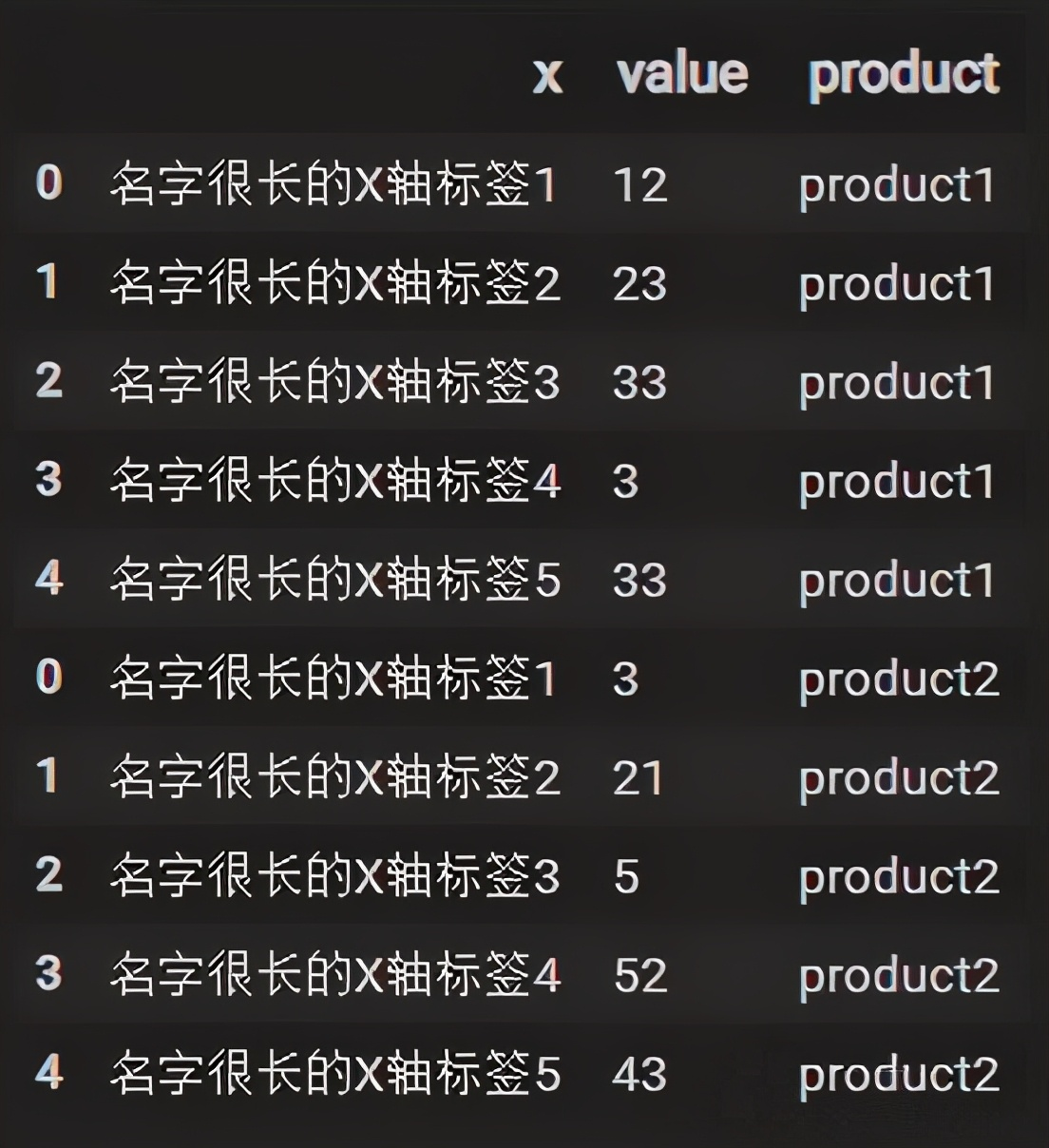# 一、matplotlib

matplotlib是学习python数据分析必定接触到的一个库，是用来绘图的基础的库，也是其他各个可视化库的基本库之一。

```import matplotlib.pyplot as plt  # 导入绘图库

# 设置绘图直接显示
%matplotlib inline

# 设置绘图的整体表现
plt.style.use('ggplot')  # 设置绘图主题为 ggplot
plt.rcParams['font.sans-serif'] = ['SimHei']  # 设置图像字体为黑体，同时可以显示中文
plt.rcParams['axes.unicode_minus'] = False  # 设置显示负号

# 设置画布大小
plt.figure(figsize=(12, 7))

# 绘制柱状图--堆积柱状图
# 绘制下面的图
plt.bar(
left = data.x.unique(), ## 设置x轴内容
height = data.value[data['product'] == 'product1'],  ## 设置y轴内容
label = 'product1'  ## 设置标签
)

# 绘制上面的图
plt.bar(
left = data.x.unique(), ## 设置x轴内容
height = data.value[data['product'] == 'product2'],  ## 设置y轴内容
bottom = data.value[data['product'] == 'product1'],  ## 设置上面的图以下面的图的数据为底
label = 'product2'  ## 设置标签
)

## 设置图例标签，标签位置为上面中部，文字大小为15，图例是平铺（图例按两列排，即在一行）
plt.legend(loc='upper center', fontsize=15, ncol=2)```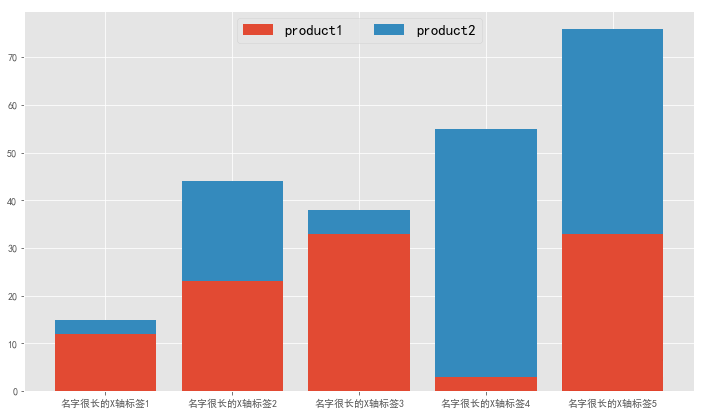# 二、seaborn

seaborn是基于matplotlib而做出来的一个可视化的封装库，相对于matplotlib的优点在于可以用比matplotlib更短的代码实现比较复杂的图像，缺点在于无法进行更强大的定制。

```from plotnine import *  ## 导入plotnine库

## 绘制前加括号
(
ggplot(data,aes(x='x',y='value',fill='product')) ## 数据对象
+ geom_bar(stat='identity',width=0.5) ## 绘制柱状图的API
+ theme(  ## 对绘图的表现进行调整
text = element_text(family = "SimHei"),  ## 设置黑体，可以显示中文
legend_direction ='horizontal',  ## 图例水平呈现
legend_title = element_blank(),  ## 图例标题不显示
legend_background = element_rect('none'),  ##  图例没有背景色
legend_position = (0.52,0.83),  ## 设置图例位置
figure_size = (9,5),  ##  画布的大小
axis_title_x = element_blank(),  ## X轴标题为空
axis_title_y = element_blank()  ## Y轴标题为空
)
)```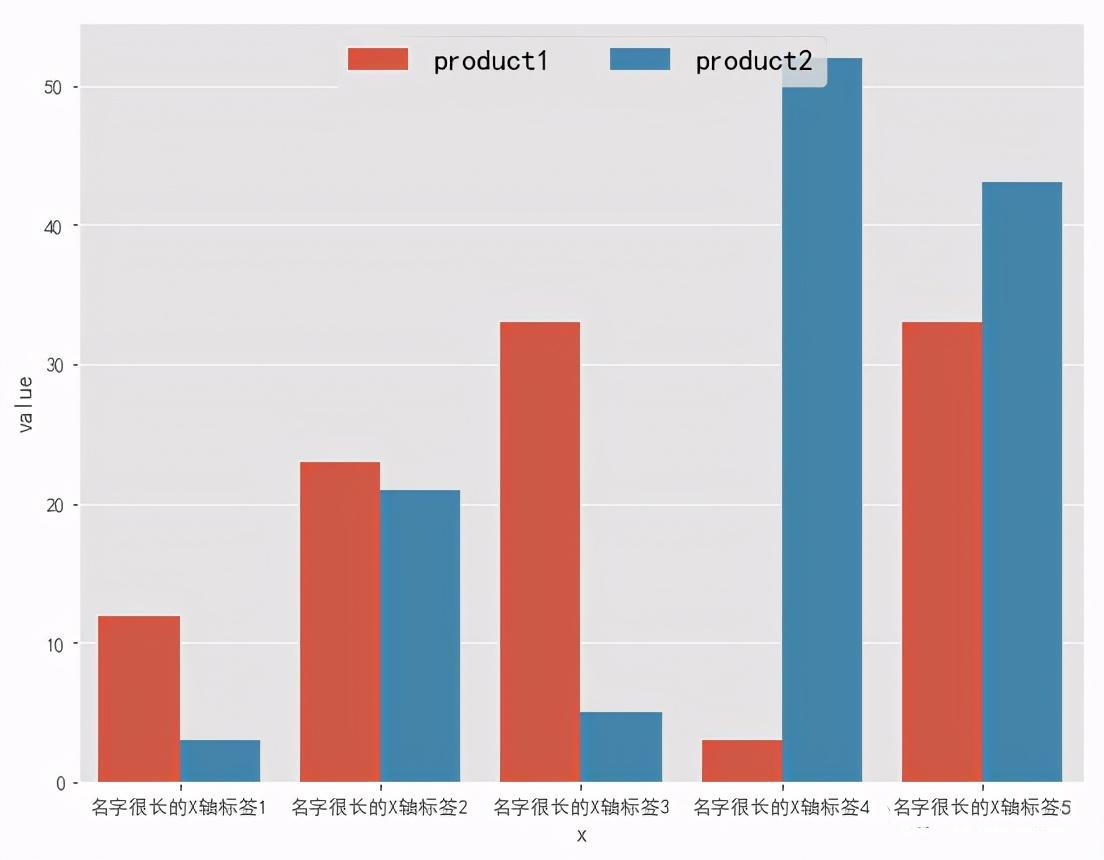# 三、plotnine

R语言的绘图在数据分析的语言里一直是比较强的，而plotnine是德国的一个大神模仿R语言里面的ggplot2，在python库里开发的一个很强大的可视化库，可以绘制出很好看的库，相对于matplotlib更易上手，也更好调试。而且更有意思的是，由于plotnine库相对小众，许多查不到方法的可以直接去查询R语言里面的一些方法，基本上也可以对接的上。

```from plotnine import *  ## 导入plotnine库

## 绘制前加括号
(
ggplot(data,aes(x='x',y='value',fill='product')) ## 数据对象
+ geom_bar(stat='identity',width=0.5) ## 绘制柱状图的API
+ theme(  ## 对绘图的表现进行调整
text = element_text(family = "SimHei"),  ## 设置黑体，可以显示中文
legend_direction ='horizontal',  ## 图例水平呈现
legend_title = element_blank(),  ## 图例标题不显示
legend_background = element_rect('none'),  ##  图例没有背景色
legend_position = (0.52,0.83),  ## 设置图例位置
figure_size = (9,5),  ##  画布的大小
axis_title_x = element_blank(),  ## X轴标题为空
axis_title_y = element_blank()  ## Y轴标题为空
)
)```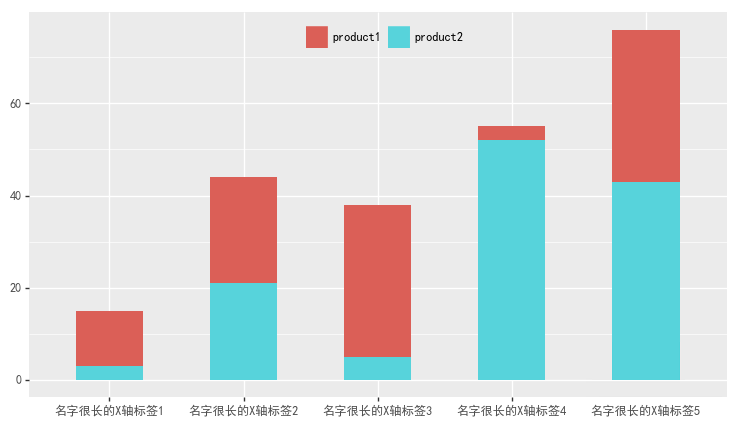plotnine可以设置很多参数，而且相对matplotlib这些参数也很多都清晰易懂，绘制出来的图也是简单大气，我在前面matplotlib的绘制加入的ggplot主题同样也是matplotlib里面模仿R语言ggplot库的。

# 四、pyecharts

Echarts是百度开源的一个可视化工具，被几个大神对接到python上面开发出了pyecharts库。这个库我现在也在学习，是个相当强大的可视化工具，不过其中有很多内容比较复杂，但是图表实现起来确实好看。

```from pyecharts import options as opts  ## 导入配置项，用来配置图像的各个表现
from pyecharts.charts import Bar  ## 导入柱状图
from pyecharts.globals import ThemeType  ## 导入主题选项，用来设置主题

c = (
Bar(init_opts=opts.InitOpts(theme=ThemeType.LIGHT)) ## 绘制柱状图，设置主题为 LIGHT
"product1",  ## 设置第1组数据图例
list(data.value[data['product']=='product1']),  ## 导入第1组数据
stack="123",  ## 设置statck
category_gap="50%"  ## 设置柱状图的宽度比例
)
"product2", ## 设置第2组数据图例
list(data.value[data['product']=='product2']),  ## 导入第2组数据
stack="123",  ## 设置statck
category_gap="50%"  ## 设置柱状图的宽度比例
)
.set_series_opts( ## 设置系列配置项
label_opts=opts.LabelOpts(is_show=False)  ## 设置数据标签不显示
)
.set_global_opts(  ## 配置全局配置项
## 由于X轴内容比较长，设置一个倾斜，解决x轴标签内容过长的问题
xaxis_opts=opts.AxisOpts(axislabel_opts=opts.LabelOpts(rotate=-15))
)
.render("stack_bar_percent.html")  ## 保存为html格式
)```

`` ``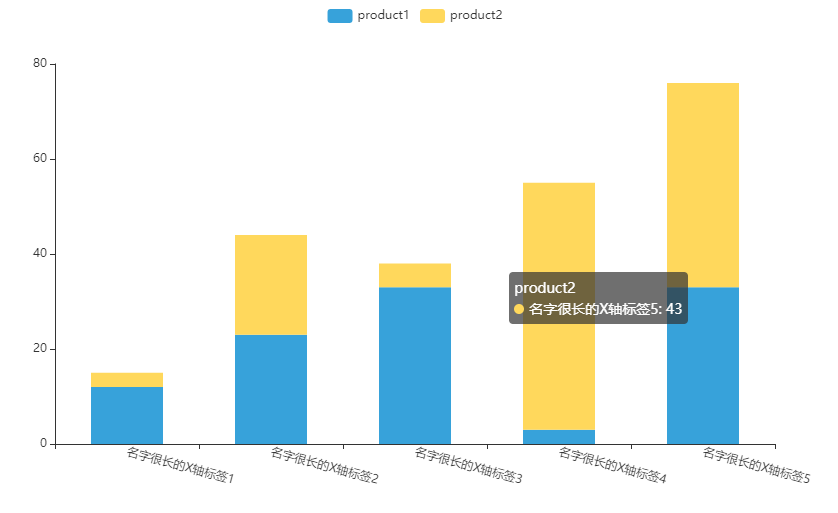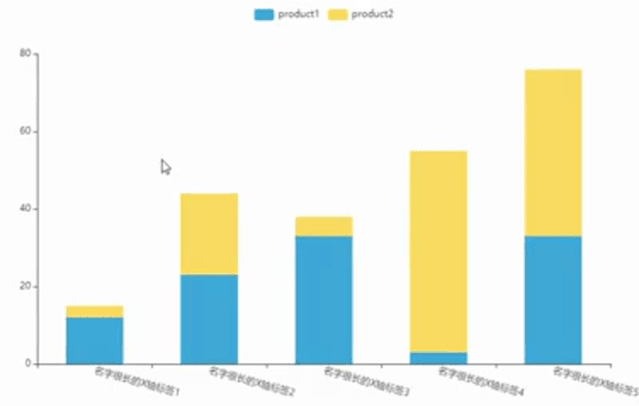pyecharts可以实现很好看的图表显示，而且配置也很全，最关键的是，pyecharts可以以动态可选择的展示。

# 五、pandas

pandas是python数据处理里最常用的一个库，学习python数据分析绝对绕不开的一个库。不仅仅是数据处理的简便，在pandas里还集成了一些可视化的接口，实现简单的数据图表。

```data2=pd.concat(
[
data[['x']][data['product']=='product1'],
data[['value']][data['product']=='product1'].rename(columns={'value':'product1'}),
data[['value']][data['product']=='product2'].rename(columns={'value':'product2'})
],axis=1
)``````data2.plot(
kind='bar', ## 设置绘图的形状为柱状图
x='x',  ## 设置x轴内容
stacked=True,  ## 设置是否为堆积柱状图
figsize=(12,7),  ## 设置画布大小
rot=15  ## 设置x轴内容角度
)

## 设置图例标签，标签位置为上面中部，文字大小为15，图例是平铺（图例按两列排，即在一行）
plt.legend(loc='upper center',fontsize=15,ncol=2)```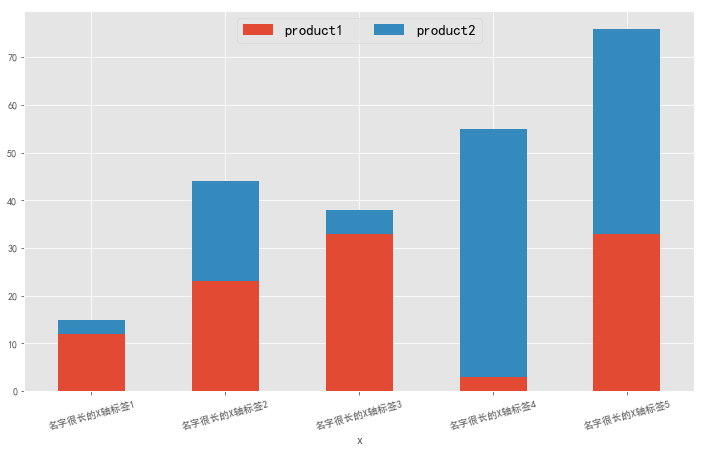pandas作图的优势就在于可以直接用pandas里的API进行作图，缺点就在于无法定制更多的可视化内容，只能做一些简单的图表。

3 0